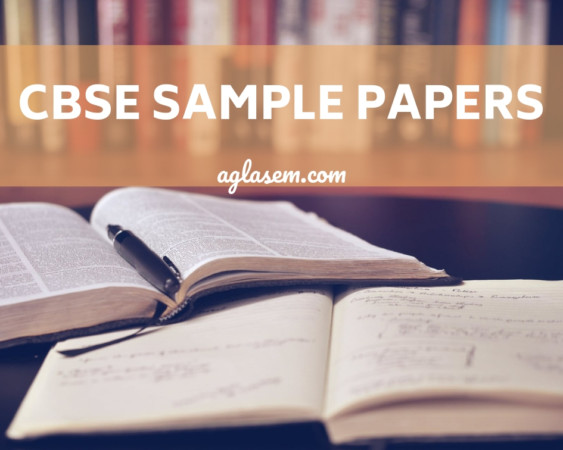CBSE Sample Question Papers for Class 11 Maths Set C with Answers are now available for download in PDF format. CBSE Sample Paper are provided below with answers as per the guidelines of CBSE board marking scheme.

Central Board of Secondary Education Board Exam Sample Question Paper for Class 11 are given here. CBSE Class 11 Maths Sample Paper gives an idea of question paper pattern and marking scheme.

## CBSE Sample Paper for Class 11 Maths (Solved) – Set C

No chapter wise weightage for Class 11 Maths Paper. Care to be taken to cover all the chapters. The above paper is only a sample. Suitable internal variants may be made for generating similar templates keeping the overall weightage to different form of questions and typology of questions same. Set C of Solved CBSE Sample Paper for Class 11 Maths is given below with its solutions.

## Sample Question Paper

Section-A

Question numbers 1 to 6 carry 1 mark each.

1. Describe the following set in roster form : {x ∈ N : x2 < 70}.

2. Given A = {1, 3, 5} and B = {2, 41. List the elements of relation R, if R = {(x,y) ∈ A x B : x > y}.

3. Find the derivative of sin2 x with respect to x.

4. The foci of an ellipse are (± 5, 0) and eccentricity is -2′ Find the equation of the ellipse.

5. Two vertices of a triangle are (2, – 6, 4), (4, – 2, 3) and its centroid is (8/3, -1, 3) Find third vertex.

6. Write the negation of the following statement :

“The number 2 is greater than 7.”

Section-B

Question numbers 7 to 19 carry 4 marks each.

7. Prove that : cos 10° cos 30° cos 50° cos 70° = 3/16

8. In a group of 40 students, 26 take tea, 18 take coffee and 8 take neither of the two. How many take both tea and coffee ?

9. Solve the trigonometrical equation : tan2 θ + (1 – √3) tanθ – √3 = 0.

10. Find the number of different 8-letter words (may or may not have a meaning) which can be formed om the letters of the word ‘EQUATION’, which start with a vowel.

OR

Find n, if P (n, 4) : P (n – 1, 3) = 9 : 1.

11. An urn contains 5 blue and an unknown number x of red balls. Two Jails are drawn at random.

If the probability of both of them being blue is 5/14 Find x.

Evaluate :OR13. If x + iy = √(a + ib)/(p + iq) Prove that : (x2 + y2)2 = (a2 + b2)/(p2 + q2)

OR

Find the value of √-16 + 30i + √-16 – 30i

14. If p and p’ be perpendiculars from the origin upon the straight lines whose equations are x sec θ + y cosec θ = a and x cos θ – y sin θ = a cos 2θ, prove that 4p2 + p2 = a2.

15. Two cards are drawn from a pack of 52 cards. What is the probability that either both are red or both are kings ?

16. Find mean deviation about median for the following data :

 C.I. 16 – 20 21-25 26 – 30 31 – 35 36 – 40 41-45 46 – 50 51-55 f 5 6 12 14 26 12 16 9

17. Draw the graph of greatest integer function.

OR

Draw the graph of Signum Function f : R → R defined by18. Find the equation of set of points `P’ such that its distance from the points (3, 4, – 5) and (- 2, 1, 4) are equal.

2+√2+√2 + 2 cos 8θ = 2 cosθ

Section-C

Question numbers 20 to 26 carry 6 marks each.

20. Prove that the diagonals of the parallelogram formed by the four straight lines :

3x + y = 0; √3y + x = 0; √3x + y = 1 and √3y + x = 1. are at right angles to one another.

21. Evaluate : (√2 + 1)6 + (√2 – 1)6

OR

Prove that there is no term involving x5 in the expansion of (2x2 – 3/x)11.

22. In any triangle ABC, prove that :
(i) (sin (B – C))/(sin (B + C)) = (b2 – c2)/a2

(ii) b cos B + c cos C = a cos (B – C). [Supplementry Material Issued by Board]

23. In ancient time the main means of communication was letters, (Post cards, International cards). People would feel so happy to write/read letters for their relatives with full emotions. But now the means of communication is very fast and popular as phones, emails, SMS etc. An aged person decides to write a letter to four of his friends. He asks each one of them to copy the letters and mail to four different persons with the instruction that they move the chain similarly. Assuming that the chain is not broken and that it cost 50 paise to mail one letter. Find the amount spent on the postage when 8th set of letters is mailed. Write any one importance of each means of communication. [Value Based Question]

24. Solve graphically : 2x + y ≥ – 3, 2x + y ≤ 6, x ≥ 0, y ≥ 0.

25. By using “Principle of Mathematical Induction”, prove that for all n ∈ N: 52n – 1 is divisible by 24.

26. Many students have habit to arrange their book shelf properly in a particular manner. A student wants to arrange 3 Mathematics, 4 Hindi and 5 Physics books on a shelf. In how many ways books can be arranged ? How many arrangements are possible if all the books on the same subject are to be together ? Assume that books on the same subject are different. State any one quality of such students. [Value Based Question]